{{ message }}

# Force Field Object Settings

Ryan Guy edited this page Sep 23, 2022 · 17 revisions

#### Technical Support

##### Clone this wiki locally

Force Field objects generate a force vector field around or near the object that can be used to direct fluid motion, typically attracting fluid towards or repelling fluid away from the object.

Related Topics

# Creating a Force Field

Creating a force field object is similar to creating other object types in the simulator (Fluid/Inflow/Outflow/Obstacle):

1. Add a Blender object to your scene.
2. Add this object is a FLIP Fluid object by clicking the FLIP Fluid operator in the physics panel.
3. Set the Type of this object to Force Field.
4. Set the Force Field Mode for this object.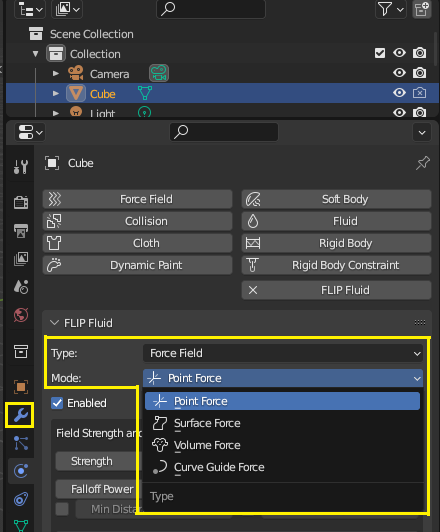## Can the built-in Blender force fields be added as a FLIP Fluid Force Field?

No, the built-in Blender force fields are not compatible with the FLIP Fluids addon. Our addon has limited access to Blender's force field system and due to this, we are not able to use these force fields within the simulator. Our force field system is a separate and custom built physics system that is implemented directly within our simulation engine.

In addition to the limitations of Blender's force field system, many of the force field modes we have implemented are for creating popular effects that are not possible using Blender's internal force fields. Our force field modes differ from Blender's in that they are specifically designed towards use in liquid physics and creating popular liquid effects.

# Parameters

## Force Field Modes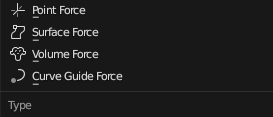Mode Type of forcefield to generate around the object. Point Force     Force field directed towards a single point. Surface Force     Force field directed towards an object's surface. Volume Force     Force field directed to fill an object's volume. Curve Guide Force     Force field directed along a curve object.

## Common Force Field Settings

Most force field modes will contain the same set of settings, even though each mode can act very differently. This section will detail the common force field settings you will find. For settings specific to an individual force field mode, see the sections below detailing each type of mode.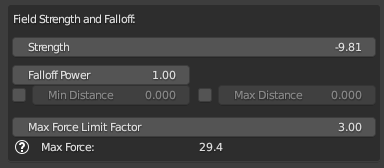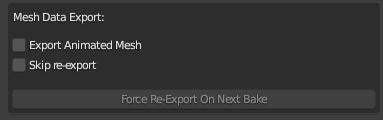Enabled Whether the force field object is active in the simulation. Strength The base strength of the force field. A negative strength attracts fluid towards the object while a positive strength will repel fluid away from the object. For reference, a strength of 9.81 will be equivalent to the default gravitational force. Falloff Power How the force strength decreases with distance away from the object. If r is the distance from the object, the force strength changes with (1 / rpower). A power of 0.0 = constant force, 1.0 = linear falloff, 2.0 = gravitational falloff. Enable Min Distance Use a minimum distance for the force field falloff. Min Distance The distance from the force object at which the force begins to falloff. The strength of the force field when the distance is less than the min distance will be (1 / min_distancepower). Only takes effect if Enable Min Distance is set to Enabled. Enable Max Distance Use a maximum distance for the force field to work. Max Distance Maximum distance from the force object that the force field will have an effect on the simulation. The strength of the force field when the distance is greater than that max distance will be 0.0. Limiting max distance can help speed up force field calculations. Only takes effect if Enable Max Distance is set to Enabled. Max Force Limit Factor The maximum force strength magnitude generated by this field will be limited to the absolute Strength value multiplied by this value. For example, if Strength = -9.81 and the Limit Factor = 3.0, the maximum strength magnitude in the field will be limited to 9.81 * 3.0 = 29.43. Max Force (Info Display) This value estimates the maximum possible force field magnitude generated by this object, taking the Max Force Limit Factor into account. Force field strengths are inversely proportional to the distance from the object and can become very large when distances decrease. Use the Max Force Limit Factor to reduce the maximum force. For reference, a value of 9.81 is equal to the default strength of gravity. Export Animated Mesh Export the force field object mesh as an animated one. TIP: Animated objects can be slower to export, so use this option only if necessary, such as for armatures or parented objects. Objects with keyframed position/rotation/scale do not require this option to be enabled. Skip re-export If enabled, the addon will skip re-exporting this mesh when starting or resuming a bake. If this mesh has not been exported or is missing files, the addon will automatically export the required files. Notes: Animated mesh export can slow down the export stage if the mesh is complex and contains a lot of geometry. Skipping mesh export for animated meshes will speed up export and help your simulation start quicker. If your animated mesh geometry or motion has changed, you will need to disable this option or force the object to export so that the addon will re-export the mesh geometry. Force Re-Export On Next Bake When enabled, this option will force the addon to re-export the object’s mesh data the next time that the user bakes or resumes the simulation. This option overrides the Skip Re-Export option. After the object has been re-exported, the force export option will be automatically disabled so that subsequent bakes will continue skipping the mesh for export. This option is only applicable if the Skip Re-Export option is enabled. TIP: Useful for if you have a complex animation or object with a lot of geometry with the Skip Re-Export option enabled to save time during export. Use this option if you make an change to your mesh geometry and want to easily mark the object to update the next time you bake/resume the simulation.

### Terminology: What does distance mean for each force field mode?

Depending on the selected force field mode, the Distance term in the above settings will have different meanings. Here is what we mean by Distance for the applicable force field modes:

• Point Force: The distance from the center of the object.
• Surface and Volume Force: The distance from the closest point on the object's surface.
• Curve Guide Force: The distance from the closest point on the object's curve.

Note: Distance values are measured in meters. By default, 1 Blender Unit is equal to 1 meter in the simulation. Distance measurements may differ if you have altered the Relative or Absolute world scaling settings in the FLIP Fluid World panel.

## Point Force

A Point Force directs fluid towards or away from a single point. Point forces are one of the quickest types of force fields to compute.

A Point Force object can be created from a Blender Empty object or a Blender Mesh object. If a mesh object is used, the origin point of the force field will use the centroid of the object's vertices. The point force mesh object may contain non-manifold geometry. We recommend using an Empty object for point forces as there are slight performance gains for these objects.

### Point Force Object Requirements

• Point force objects can be either Blender Empty type or Mesh type objects. If a mesh object is used, the origin point of the force field will use the centroid of the object's vertices.
• Unlike other simulation objects, the mesh object may contain non-manifold geometry or dynamic topology.
• We recommend using an Empty type object for point forces as there are slight performance gains for these objects.

### Point Force Parameters

Parameters that are specific to the Point Force Field mode. For settings that are common to all force fields, refer to the Common Force Field Settings section.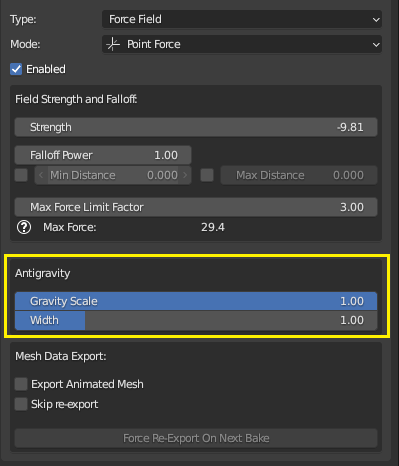Gravity Scale Scale the force of gravity around this point by this value. A scale of 0.0 is zero-gravity while a value of 1.0 will be the full gravity strength. Gravity Scale Width The maximum distance around this point where gravity scaling will take effect. The gravity scale will transition linearly by distance. At a distance of 0.0, the gravity will be scaled by the Gravity Scale value, and up until a distance of Width, the gravity will be scaled linearly up to the full strength of 1.0.

## Surface Force

A Surface Force directs fluid towards or away from the surface of an object in the direction perpendicular to the surface. Surface forces are one of the more expensive types of force fields to compute.

### Surface Force Object Requirements

• Surface force objects are required to be Blender mesh type objects.
• Unlike other simulation objects, the mesh object may contain non-manifold geometry or dynamic topology. This includes planar meshes.

### Surface Force Parameters

Parameters that are specific to the Surface Force Field mode. For settings that are common to all force fields, refer to the Common Force Field Settings section.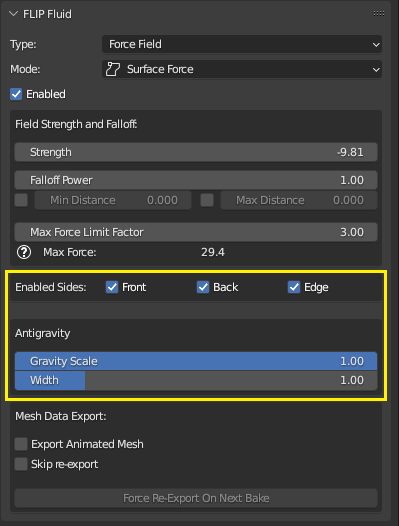#### Enabled Sides

 Front Enable force field on the front-facing polygons. This is the side where face normals point outwards. Back Enable force field on the back-facing polygons. This is the side opposite of the face normal. Edge Enable force field on planar edges. These are the edges of the surface that are not connected to any other polygons. Must have either one of Back or Front sides enabled for edge attraction to be enabled. Tip: Disable this option if you want the fluid to be attracted to the surface while still being able to flow off the edges of the surface. This option is similar to the Curve Guide Force object Endcaps option.

#### Anti Gravity

 Gravity Scale Scale the force of gravity around the surface of the mesh by this value. A scale of 0.0 is zero-gravity while a value of 1.0 will be the full gravity strength. Gravity Scale Width The maximum distance from the surface of the mesh where gravity scaling will take effect. The gravity scale will transition linearly by distance. At a distance of 0.0, the gravity will be scaled by the Gravity Scale value, and up until a distance of Width, the gravity will be scaled linearly up to the full strength of 1.0.

### Tips for fluid adhesion

Getting fluids to stick effectively to an object's surface can be difficult in fluid simulation. Use an obstacle object in combination with a surface force object to help the fluid stick to an object. Setting the Gravity Scale value to 0.0 for a small width around the force field will negate gravity near the surface, which can help further aid fluid adhesion.

See our Fluid Adhesion Example Scene for an example simulation setup.

## Volume Force

A Volume Force directs fluid towards the interior of the object's volume in the direction perpendicular to the object's surface. Volume forces are one of the more expensive types of force fields to compute.

### Volume Force Object Requirements

• Volume Force objects are required to be Blender mesh type objects.
• Object geometry is required to be Manifold/Closed/Watertight in order to work correctly and predictably within the FLIP Fluids simulator. The objects are required to have volume and planar surfaces cannot be used.
• The object should be sufficiently large/thick in order to be resolved on the simulation grid. The smallest/thinnest geometry features of the object should cover at least one voxel on the simulation grid (What is the simulation grid?).
• Unlike other simulation objects, this mesh object is allowed to contain changing topology between frames.

### Volume Force Parameters

Parameters that are specific to the Volume Force Field mode. For settings that are common to all force fields, refer to the Common Force Field Settings section.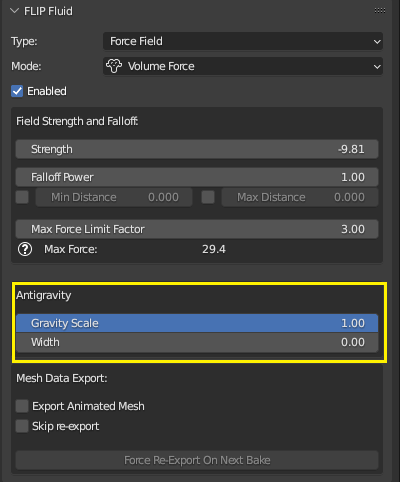Gravity Scale Scale the force of gravity inside of the mesh by this value. A scale of 0.0 is zero-gravity while a value of 1.0 will be the full gravity strength. Gravity Scale Width The maximum distance from the outside of the mesh's surface where gravity scaling will take effect. The gravity scale will transition linearly by distance. At a distance of 0.0, the gravity will be scaled by the Gravity Scale value, and up until a distance of Width, the gravity will be scaled linearly up to the full strength of 1.0.

### Fluid Control Objects

Fluid Control Objects were a feature in the older Blender Elbeem simulator that could be used to create 3D fluid shapes. Since Blender 2.82+, this feature has been removed. Our FLIP Fluids volume force can be used to mimic and improve on the older control object feature.

Here are some tips on how to set up a volume force to behave similarly to a fluid control object:

• Enable a thin Max Distance value so that the force field only affects fluid that is close to the object. Fluid further away from the object will be unaffected by the force field and will flows naturally with the regular scene gravity.
• Set the Gravity Scale to a low value, such as 0.1. This reduces the gravity inside of the force field to help the fluid hold it's shape and stay inside of the object.
• Set the Gravity Scale Width to a thin layer, such as 0.25. This results in the gravity scale extending outside of the object's surface, which can help draw fluid into the inside of the object.

See our Fluid Control Object Example Scene for an example simulation setup.

See the BlenderPhysics video for a tutorial on how to use volume forces to transform liquid between different shapes: Liquid Transformations Tutorial using FLIP Fluids Addon.

## Curve Guide Force

A Curve Guide Force directs fluid along a curve. Curve forces can require a significant amount of computation, but are less intensive compared to Surface Forces and Volume Forces.

### Curve Guide Force Object Requirements

• Curve Guide Force objects are required to be Blender Curve type objects.
• The curve object may be either open-ended or a closed-loop.
• The curve object should only consist of a single continuous curve. If the object is made up of multiple separate curves, only the first curve will be used in the simulation. Multiple curves should be separated into multiple objects.
• Unlike other simulation objects, the curve object may change topology between frames.

### Curve Force Parameters

Parameters that are specific to the Curve Force Field mode. For settings that are common to all force fields, refer to the Common Force Field Settings section.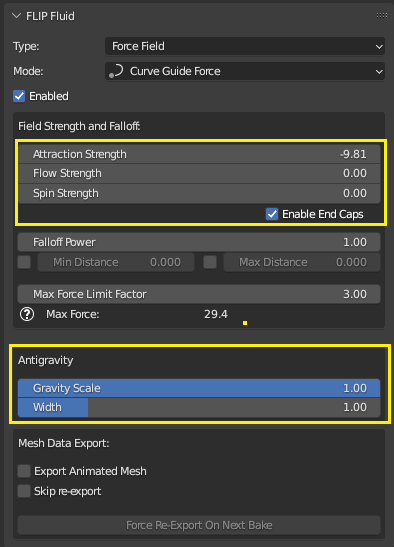Strength The base strength of the force field. A negative strength attracts fluid towards the closest point on the curve while a positive strength will repel fluid away from the curve. For reference, a strength of 9.81 will be equivalent to the default gravitational force. Flow Strength The strength of of the force that propels fluid along the curve. A positive strength will direct fluid in the direction from the first curve vertex to the last curve vertex. A negative strength will reverse the direction. Spin Strength The strength of of the force that propels fluid in a vortex around the curve. A positive strength spin direction uses the Right Hand Rule: take your right hand an point your thumb in the direction of the curve (fist vertex towards last vertex). Curling your right-hand fingers around the curve will be the direction of spin. A negative strength will reverse the spin direction. Enable End Caps Whether fluid will be attracted to the end points of the curve segment. Disable to allow fluid to flow past the end of the end points of the curve segment. Gravity Scale Scale the force of gravity near the curve by this value. A scale of 0.0 is zero-gravity while a value of 1.0 will be the full gravity strength. Gravity Scale Width The maximum distance from the closest point on the curve where gravity scaling will take effect. The gravity scale will transition linearly by distance. At a distance of 0.0, the gravity will be scaled by the Gravity Scale value, and up until a distance of Width, the gravity will be scaled linearly up to the full strength of 1.0.

### Notes on Flow and Spin Strength

Due to the nature of how the physics of force fields work, fluid under the influence of a force will accelerate (speed up) over time, similar to how an object under the force of gravity will speed up the longer it falls. A Flow Strength force will cause the fluid to accelerate in the dircetion of the curve. A Spin Strength force will cause the fluid to accelerate around the curve and spin faster. If acceleration for Flow and Spin forces are not desired, you may want to use their strength for a short period of time, such as by keyframing the strength down to 0.0.

### Creating a Vortex Using a Curve Guide Force Field

The curve guide force can also be used to create a vortex-like force field. This can be done by using a curve that is a straight line while using a spin strength to have the fluid flow in a vortex pattern. Our Stairstep Ocean example scene file contains a portion showing how this works in the scene notes and setup.

For a video guide on creating a swirling vortex simulation, see this tutorial by Pixel Planet Studios: Intro to FLIP Fluids - Part 1: Getting Started (timestamp: 7:40).